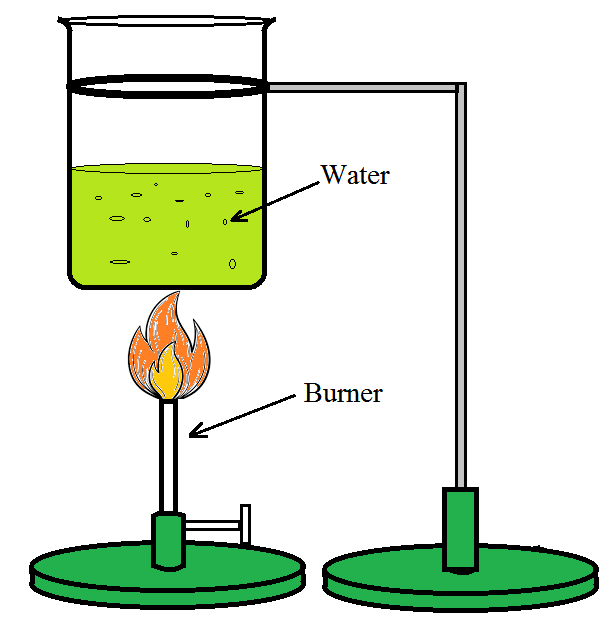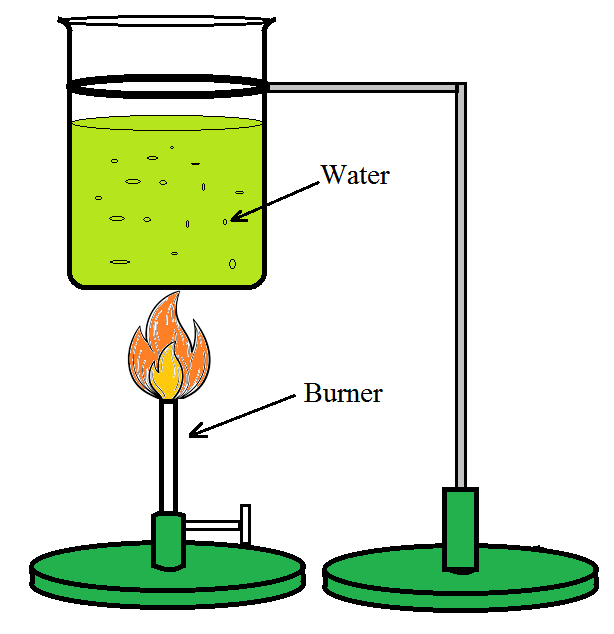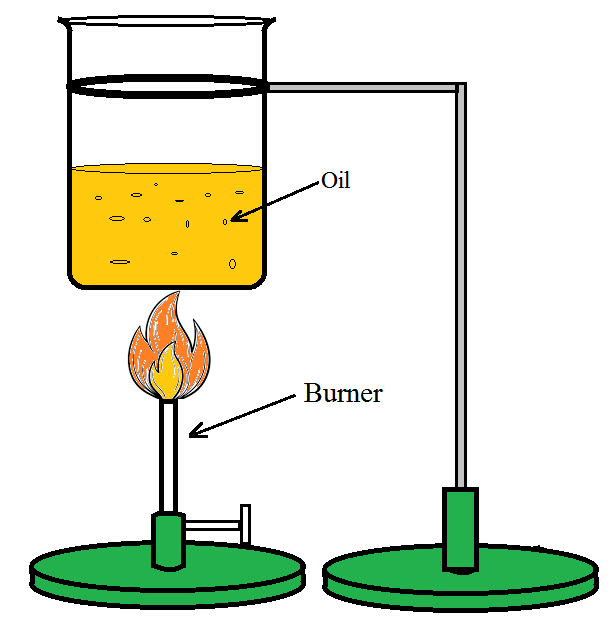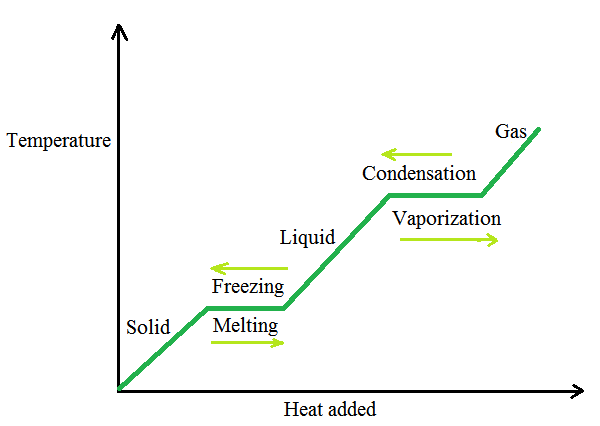Heat Capacity

• Last Updated : 30 Jun, 2021

Thermal properties of matter cause a matter to conduct heat or that determine the nature of the matter in the presence of heat. As a result, when heat passes through an object, it exhibits thermal properties. Different materials or things behave differently under the impact of heat according to their thermal characteristics. Alternatively, these qualities determine how matter reacts when subjected to heat changes.

Thermal characteristics are divided into four categories: Heat capacity, Thermal Expansion, Thermal conductivity, and Thermal stress.

Let’s see some examples fill a vessel halfway with water and place it on a stove to heat. The bubbles start to rise. As the temperature increases, the velocity of water particles is increased, and it becomes turbulent as the water begins to boil.

• The first stage is to heat a specified amount of water to a temperature of, say, 20 degrees Celsius, and record the time it takes. Using the same source of heat, raise the temperature of the same amount of water by 40 degrees Celsius and record the time. It is observed that it took nearly twice the time so, double the amount of heat required to raise the temperature of the same amount of water.Figure 1

• Now in the second step take double the quantity of water and heat it using the same heating system to raise the temperature by 20 °C, it is observed that the time necessary will be twice that of the first stage.Figure 2

• In the third stage, heat the same amount of oil, in place of the water, and raise the temperature by 20 degrees Celsius. Record the time. It is observed that the time it takes is shorter, and as a result, the amount of heat required is less than that required by the same volume of water for the same temperature rise.Figure 3

What is Heat Capacity?

The change in temperature of a substance, when a given quantity of heat is absorbed or rejected by it, is characterized by a quantity called the heat capacity of that substance.  It is denoted by S.

It is expressed as,

S= ΔQ / ΔT

where ΔQ is the amount of heat supplied to the substance to change its temperature from T to T + ΔT

Specific heat capacity

When the same quantity of heat is applied to the same mass of different substances, the resulting temperature changes are not the same. It indicates that each substance has a distinct value for the quantity of heat absorbed or rejected to change the temperature of its unit mass by one unit. The specific heat capacity of a substance is the measurement of this quantity. It is symbolized by the letter s.

If S the amount of heat absorbed or rejected i.e. ΔQ by a substance of mass m when it experiences a temperature change ΔT, then the specific heat capacity, of that substance is given by

s = S/m = (1/m) (ΔQ / ΔT)

Specific heat capacity is defined as the number of heat changes i.e. heat absorbed or rejected by a substance per unit mass in order to change its temperature by one unit.

The specific heat capacity is the property of the substance which determines the change in temperature of the given quantity of a substance that is the amount of heat is absorbed or rejected.

The given substance is undergoing no phase change during temperature change. It is dependent on the substance’s nature and temperature.

J kg–1 K–1 is the SI unit for specific heat capacity.

Molar specific heat capacity

If the amount of substance is provided in moles rather than mass m in kilograms then the heat capacity per mole μ of a substance can be calculated as follows:

C = S/μ = (1/μ)(ΔQ/ ΔT)

where C denotes the substance’s molar specific heat capacity. It also depends on the nature and temperature of the substance.

The SI unit is Jmol–1 K–1.

The molar specific heat capacity at a fixed pressure, denoted by Cp, is the molar specific heat capacity at fixed pressure when the gas is held at fixed pressure during the heat transfer. If the volume of the gas is kept fixed during the heat transfer, the corresponding molar specific heat capacity is known as molar specific heat capacity at constant volume and is indicated by Cv.

When compared to other substances, water has the highest specific heat capacity at atmospheric pressure. That is why water is utilized as a coolant in automotive radiators and as a heater in hot water bags. During the summer, the water warms up much more slowly than the land due to its large specific heat capacity, and as a result, the wind from the sea has a cooling impact.

Let’s also discuss other thermal characteristics as:

Thermal conductivity:

Not all materials are capable of transferring heat through their bodies. Conductors are those who can. As a result of their thermal conductivity, such materials allow heat to passes through them. There are conductors with a high conductivity, which means they conduct more heat than those with a low conductivity of heat. Insulators are materials that do not conduct heat in any way.

Thermal stress:

Metals have the ability to transport or transfer heat as one of their qualities. This transfer results in physical changes like expansion when the temperature rises and contraction when the temperature falls. This occurs in each of the three dimensions. When the temperature changes, the thermal expansion of metallic structural members causes thermal stress. Thermal deformation of structural members is caused by temperature changes.

Thermal stress has the ability to harm a thing in some cases, hence it could be destructive in nature. For example, large truck tires develop cracks. This is because high-speed driving causes heat to be produced by friction between the road’s surface and the tires. The tires crack as a result of the resulting heat stress.

Temperature and Heat:

Temperature is a measure of hotness or coolness that is relative. A hot utensil has a high temperature, whereas an ice cube has a low temperature. A hotter object is one that has a higher temperature than another one. Hot and cold, like tall and short, are relative concepts.

On a hot summer day, a glass of chilled water left on a table slowly warms up, whereas a cup of hot coffee on the same table cools down. When the temperature of the body, in this case, chilled water or hot coffee, and the temperature of the surrounding medium differ, heat transfer occurs between the system and the surrounding medium continues till the body and the surrounding medium are coming at the same temperature.

Heat flows from the environment to a glass tumbler of chilled water, but it flows from the cup of hot coffee to the environment in the case of hot coffee. As a result, it is defined heat as the form of energy exchanged through temperature differential between two (or more) systems or between a system and its surroundings.

The SI unit of heat energy transported is the joule (J), while the SI unit of temperature is the Kelvin denoted by K, and the frequently used unit of temperature is the degree Celsius denoted by °C. Many changes can occur when an object is heated. Its temperature can alter, it can grow, and it can change states.

Change of State:

Solid, liquid, and gas are the three basic states of matter. A change of state is the movement from one of these states to another. Solid to liquid and liquid to gas are two common state transitions (and vice versa). When heat is exchanged between the substance and its surroundings, certain changes can occur.

Melting is the transformation of a solid into a liquid, whereas fusing is the transformation of a liquid into a solid. During the transition from solid to liquid, the substance’s solid and liquid phases coexist in thermal equilibrium. The melting point of a substance is the temperature at which its solid and liquid states are in thermal equilibrium with one another. It’s a property of the substance. It also depends on the amount of pressure. The normal melting point of a substance is its melting point at standard atmospheric pressure.

The transition from a liquid to a vapor (or gas) is known as vaporization. The temperature is found to remain constant until the full amount of liquid is turned to vapor. That is, throughout the transition from liquid to vapor, the substance’s liquid and vapor states coexist in thermal equilibrium. The temperature at which a substance’s liquid and vapor states coexist is known as its boiling point.

Latent Heat

The heat or energy absorbed or released during a phase shift of a substance is known as latent heat.

It could be from a gas to a liquid or from a liquid to a solid. Enthalpy is a heat attribute that is related to latent heat. However, one crucial factor to remember about latent heat is that the temperature of the substance does not change. In terms of the process, latent heat is the effort required to overcome the attractive forces that hold molecules and atoms in a substance together.At the boiling point, a liquid changes its state. When you add extra heat to boiling water, it vaporizes without increasing the temperature. The amount of heat required during a change of state is determined by the heat of transformation and the mass of the substance changing states. As a result, if the mass m of a substance changes from one state to another, the amount of heat required is given by

Q = m L

or

L =Q/m

L stands for latent heat, which is a property of the substance. J kg–1 is its SI unit. L’s value is likewise affected by the pressure. Its value is often expressed in terms of ordinary atmospheric pressure. The latent heat of fusion (Lf) is used to describe a solid-liquid state change, while the latent heat of vaporization (Lv ) is used to describe a liquid-gas state change.

Sample problems

Problem 1: A 88.3 g sample of metal at 95.24  C is added to 35.10 g of water that is initially at 17.27 °C. The final temperature of both the water and the metal is 29.20 °C. The specific heat of water is 4.184 J/(g°C). Calculate the specific heat of the metal.

Solution:

Given,

Mass of metal is 88.3 g

The initial temperature of the metal is 95.24 °C.

Mass of water: 35.10 g.

The initial temperature of the water is 17.27 °C.

The final temperature of the water and the metal is 29.20 °C.

The specific heat of water is  4.184 J/(g°C).

Therefore, the expression where the energy from the hotter metal transfers to the cooler water is

−moCoΔTo=mwCwΔTw

Where

mo= mass of a metal object

ΔTo = temperature change of metal object

Co= specific heat capacity of metal object

mw= mass of water

ΔTw= temperature change of water

Cw= specific heat capacity of water

Rearrange the above expression,

Co=(mwCwΔTw)/(moΔTo)

Substitute the values in the above expression,

Co=[35.10 4.184(29.20−17.27)]/[88.3(29.20-95.24)]

Co=0.301 J/g°C

Problem 2: Define heat capacity and write its expression.

Solution:

The change in temperature of a substance, when a given quantity of heat is absorbed or rejected by it, is characterized by a quantity called the heat capacity of that substance.  It is denoted by S.

It is expressed as,

S= ΔQ/ ΔT

where ΔQ is the amount of heat supplied to the substance to change its temperature from T to T + ΔT

Problem 3: The specific heat of water is 4.18 J/(g°C). Calculate the molar heat capacity of water. Express your answer to three significant figures and include the appropriate units.

Solution:

The specific heat of water is 4.18 J/(g°C).

The expression to convert gram into mole is

4.18 J/gC x (18.0 g / mole) = 75.24 J/mole C

Hence, the molar heat capacity of water is 75.24 J/mole C

Problem 4: Define specific heat capacity and write its expression.

Solution:

When the same quantity of heat is applied to the same mass of different substances, the resulting temperature changes are not the same. It indicates that each substance has a distinct value for the quantity of heat absorbed or rejected to change the temperature of its unit mass by one unit. This quantity is referred to as the specific heat capacity of the substance. It is denoted by s.

If ΔQ stands for the amount of heat absorbed or rejected by a substance of mass m when it undergoes a temperature change ΔT, then the specific heat capacity, of that substance is given by

s = S/m = (1/m)(ΔQ/ ΔT)

The SI unit for specific heat capacity is Jkg–1 K–1 .

Problem 5: Define molar-specific heat capacity and write its expression.

Solution:

If the amount of substance is provided in moles rather than mass m in kilograms then the heat capacity per mole μ of a substance can be calculated as follows:

C = S/μ = (1/μ)(ΔQ/ ΔT)

where C denotes the substance’s molar specific heat capacity. It also depends on the nature and temperature of the substance and its SI unit is  Jmol–1 K–1.

The molar specific heat capacity at fixed   pressure, denoted by Cp, is the molar specific heat capacity at fixed pressure when the gas is held at fixed pressure during the heat transfer. If the volume of the gas is kept fixed during the heat transfer, the corresponding molar specific heat capacity is known as molar specific heat capacity at constant volume and is indicated by Cv.

Problem 6: A 30.5 g sample of an alloy at 93.0 °C is placed into 50.0 g of water at 22.0 °C in an insulated coffee cup with a heat capacity of 9.2 J/K. If the final temperature of the system is 31.1 °C, what is the specific heat capacity of the alloy?

Solution:

Heat absorbed = heat lost

then the specific heat capacity, of that substance is given by

s = (1/m)(ΔQ/ ΔT)

Rearrange the above expression,

ΔQ=smΔT

ΔQalloy = ΔQwater+ΔQcup

The temperature of the water is equal to the temperature of the cup = 22.0 °C.

The temperature of the alloy is  93.0 °C.

The final temperature is 31.1 °C.

30.5×(93.0 – 31.1)s = 9.2×(31.1-22.0) + 50.0×4.2×(31.1-22.0)

1887.95s = 1994.72

s = 1.057 J/gK

My Personal Notes arrow_drop_up